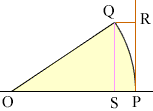Quandaries and Queries Who is asking: Student Level: Secondary Question: I'm trying to build a route in a train simulator program. I have a curve to the right (an arc, in other words) with a length of 25 meters and the radius is 1,500 meters. Let's say point P is the beginning of the curve (arc) and point Q is the end point of the arc. Then picture a tangent to point P. I need to find the length of a line perpendicular to that tangent that runs to point Q (the end of the curve/arc). The reason is because the way the coding of routes for the program works is, you control the course of your track, but everything else (including other tracks) is placed relative to your track, and I need to figure out the horizontal displacement of the track at the end of the curve from the z-axis (z-axis is forwards/backwards in this case, it is a 3-dimensional environment.) Hi, I drew a diagram of what I understand to be the problem.The problem is to find the distance QR where the line segment QR is perpendicular to the line PR.I let O be the center of the circle that defines the arc and I drew QS perpendicular to OP. The relationship between theta, the angle QOP and a, the arclength PQ is a = rtheta Here theta is measured in radians. From the diagram cos(theta) = |OS|/|QO| The unknown distance |QR| is then |QR| = |OP| - |OS| = |OP| - |QO|cos(theta) With the numbers you supplied, |OP| = |QO| = 1500 meters and the arclength PQ 25 meters I get |QR| = 1500 - 1500cos(0.016667) = 1500 - 1499.79 = 0.21 meters Penny Go to Math Central